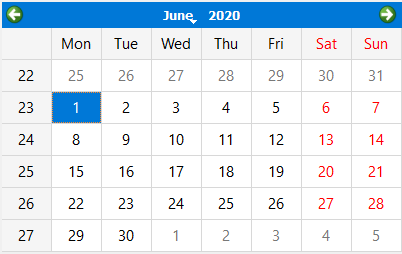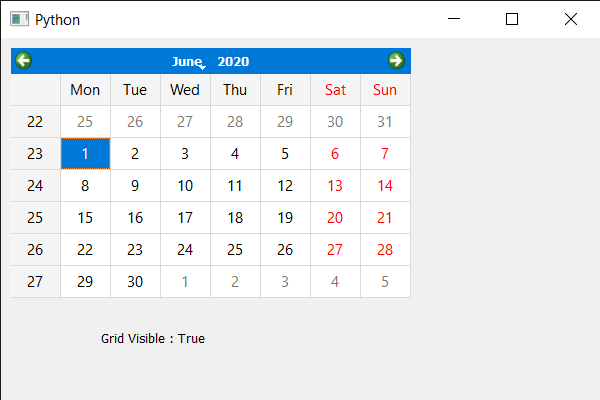Related Articles
PyQt5 QCalendarWidget – Checking if grid is visible
• Last Updated : 03 Jun, 2020

In this article we will see how we can check if grid is visible in the QCalendarWidget. Grid is basically the inter connected network of lines that separates the dates with each other. By default there is no grid to the QCalendarWidget although we can set grid any time with the help of `setGridVisible` method. Below is how calendar with grid looks like.In order to do this we will use `isGridVisible` method with the QCalendarWidget object.

Syntax : calender.isGridVisible()

Argument : It takes no argument

Return : It return bool

Below is the implementation

 `# importing libraries``from` `PyQt5.QtWidgets ``import` `*` `from` `PyQt5 ``import` `QtCore, QtGui``from` `PyQt5.QtGui ``import` `*` `from` `PyQt5.QtCore ``import` `*` `import` `sys`` ` ` ` `class` `Window(QMainWindow):`` ` `    ``def` `__init__(``self``):``        ``super``().__init__()`` ` `        ``# setting title``        ``self``.setWindowTitle(``"Python "``)`` ` `        ``# setting geometry``        ``self``.setGeometry(``100``, ``100``, ``600``, ``400``)`` ` `        ``# calling method``        ``self``.UiComponents()`` ` `        ``# showing all the widgets``        ``self``.show()`` ` `    ``# method for components``    ``def` `UiComponents(``self``):`` ` `        ``# creating a QCalendarWidget object``        ``calender ``=` `QCalendarWidget(``self``)`` ` `        ``# setting geometry to the calender``        ``calender.setGeometry(``10``, ``10``, ``400``, ``250``)`` ` `        ``# setting grid``        ``calender.setGridVisible(``True``)`` ` `        ``# creating label``        ``label ``=` `QLabel(``self``)`` ` `        ``# setting geometry to the label``        ``label.setGeometry(``100``, ``270``, ``250``, ``60``)`` ` `        ``# making label multi line``        ``label.setWordWrap(``True``)`` ` `        ``# checking if the calendar has grid``        ``check ``=` `calender.isGridVisible()`` ` `        ``# setting text to the label``        ``label.setText(``"Grid Visible : "` `+` `str``(check))`` ` ` ` `# create pyqt5 app``App ``=` `QApplication(sys.argv)`` ` `# create the instance of our Window``window ``=` `Window()`` ` `# start the app``sys.exit(App.``exec``())`

Output :Attention geek! Strengthen your foundations with the Python Programming Foundation Course and learn the basics.

To begin with, your interview preparations Enhance your Data Structures concepts with the Python DS Course.

My Personal Notes arrow_drop_up